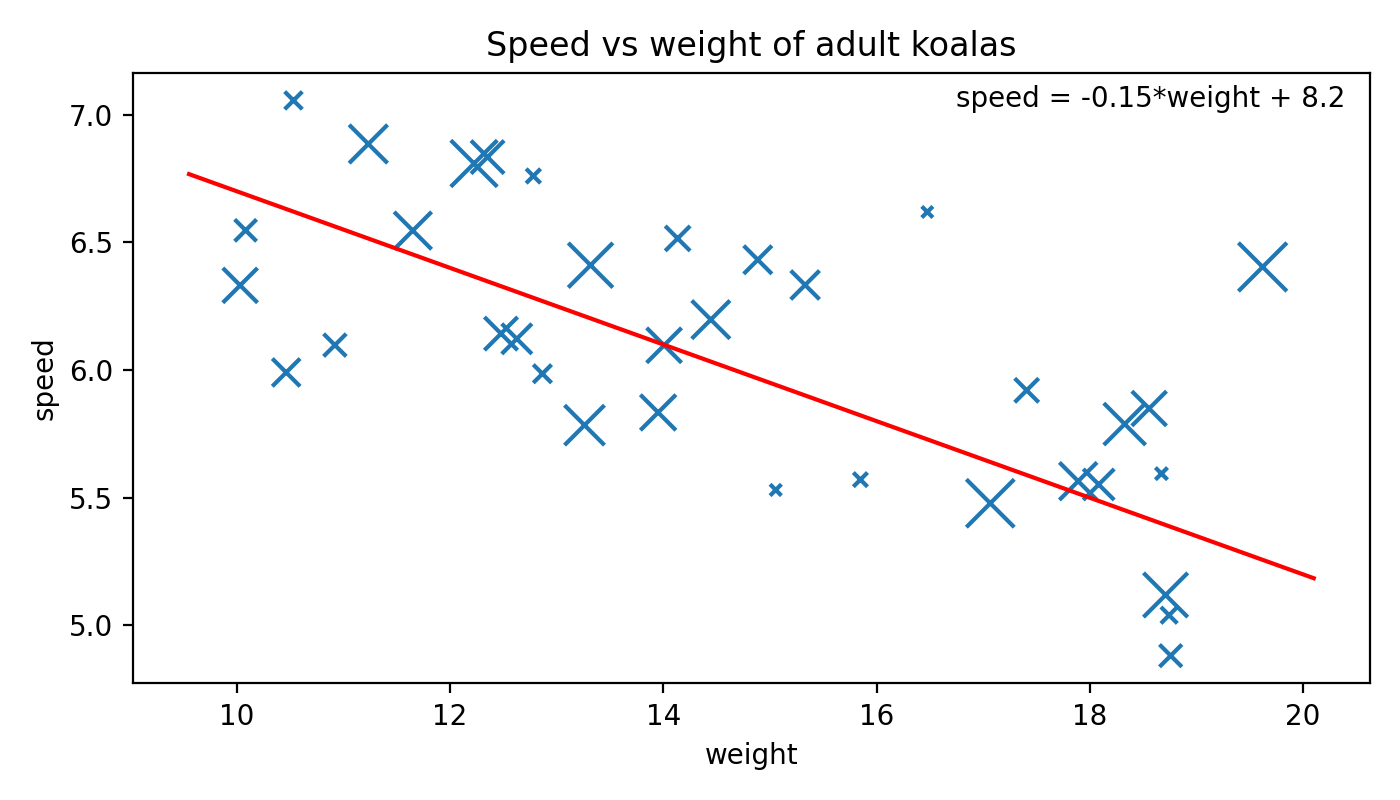# Koala Speeds Problem¶

You believe there's a linear relationship between the weight of an adult koalaand how fast it runs. You gather some data.

import numpy as np

# Create a random number generator
rng = np.random.default_rng(4321) # (1)!

# Make data
weights = rng.uniform(low=10, high=20, size=35)
speeds =  -0.08* weights + 7.2 + rng.normal(loc=0, scale=0.5, size=len(weights))
ages = rng.uniform(low=3, high=18, size=len(weights))

print(weights)  # [10.03 18.08 15.33 ... 17.07 13.32 10.08]
print(speeds)   # [6.33 5.55 6.33 ... 5.48 6.41 6.55]
print(ages)     # [12.51 11.16 10.36 ... 17.24 16.06  7.89]


You fit a linear regression model to this data, y = mx + b, with slope m = -0.15 and offset b = 8.2.

• Make a scatter plot of speed (y) vs weight (x), varying the size of each point by the koala's age.
• Overlay the linear regression line defined by m and b.
• Include the linear regression equation inside the Axes, near the top right. (Make the position of the equation independent of the data!)
Show the plot